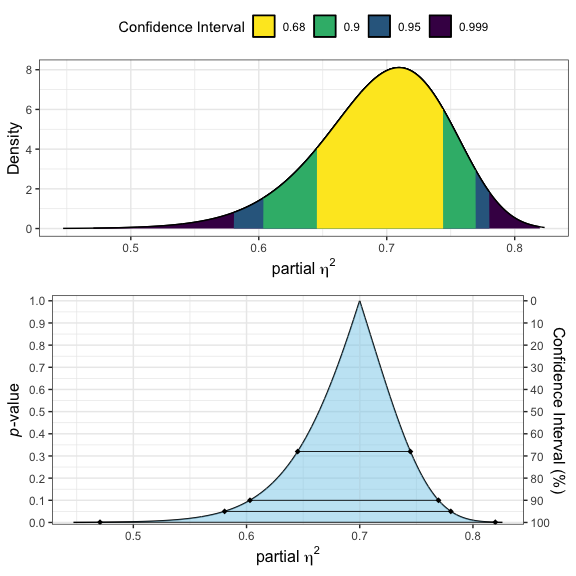# Equivalence Testing for F-tests

#### 2023-04-05

For an open access tutorial paper explaining how to set equivalence bounds, and how to perform and report equivalence for ANOVA models see Campbell and Lakens (2021). These functions are meant to be omnibus tests, and additional testing may be necessary1.

# F-test Calculations

Statistical equivalence testing (or “omnibus non-inferiority testing” as stated by Campbell and Lakens (2021)) for F-tests are special use case of the cumulative distribution function of the non-central F distribution.

As Campbell and Lakens (2021) state, these type of questions answer the question: “Can we reject the hypothesis that the total proportion of variance in outcome Y attributable to X is greater than or equal to the equivalence bound $$\Delta$$?”

$H_0: \space 1 > \eta^2_p \geq \Delta \\ H_1: \space 0 \geq \eta^2_p < \Delta$

In TOSTER we go a tad farther and calculate a more generalization of the non-centrality parameter to allow for the equivalence test for F-tests to be applied to variety of designs.

Campbell and Lakens (2021) calculate the p-value as:

$p = p_f(F; J-1, N-J, \frac{N \cdot \Delta}{1-\Delta})$

However, this approach could not be applied to factorial ANOVA and the paper only outlines how to apply this approach to a one-way ANOVA and an extension to Welch’s one-way ANOVA.

However, the non-centrality parameter (ncp = $$\lambda$$) can be calculated with the equivalence bound and the degrees of freedom:

$\lambda_{eq} = \frac{\Delta}{1-\Delta} \cdot(df_1 + df_2 +1)$

The p-value for the equivalence test ($$p_{eq}$$) could then be calculated from traditional ANOVA results and the distribution function:

$p_{eq} = p_f(F; df_1, df_2, \lambda_{eq})$

## An Example

Using the InsectSprays data set in R and the base R aov function we can demonstrate how this omnibus equivalence testing can be applied with TOSTER.

From the initial analysis we an see a clear “significant” effect (the p-value listed is zero but it just very small) of the factor spray. However, we may be interested in testing if the effect is practically equivalent. I will arbitrarily set the equivalence bound to a partial eta-squared of 0.35 ($$H_0: \eta^2_p > 0.35$$).

library(TOSTER)
# Get Data
data("InsectSprays")
# Build ANOVA
aovtest = aov(count ~ spray,
data = InsectSprays)

# Display overall results
knitr::kable(broom::tidy(aovtest),
caption = "Traditional ANOVA Test")
term df sumsq meansq statistic p.value
spray 5 2668.833 533.76667 34.70228 0
Residuals 66 1015.167 15.38131 NA NA

We can then use the information in the table above to perform an equivalence test using the equ_ftest function. This function returns an object of the S3 class htest and the output will look very familiar to the the t-test. The main difference is the estimates, and confidence interval, are for partial $$\eta^2_p$$.

equ_ftest(Fstat = 34.70228,
df1 = 5,
df2 = 66,
eqbound = 0.35)
##
##  Equivalence Test from F-test
##
## data:  Summary Statistics
## F = 34.702, df1 = 5, df2 = 66, p-value = 1
## 95 percent confidence interval:
##  0.5806263 0.7804439
## sample estimates:
##  0.724439

Based on the results above we would conclude there is a significant effect of “spray” and the differences due to spray are not statistically equivalent. In essence, we reject the traditional null hypothesis of “no effect” but accept the null hypothesis of the equivalence test.

The equ_ftest is very useful because all you need is very basic summary statistics. However, if you are doing all your analyses in R then you can use the equ_anova function. This function accepts objects produced from stats::aov, car::Anova and afex::aov_car (or any anova from afex).

equ_anova(aovtest,
eqbound = 0.35)
##        effect df1 df2  F.value       p.null      pes eqbound     p.equ
## 1 spray         5  66 34.70228 3.182584e-17 0.724439    0.35 0.9999965

### Visualize partial $$\eta^2$$

Just like the standardized mean differences, TOSTER also has a function to visualize $$\eta^2_p$$.

The function, plot_pes, operates in a fashion very similar to equ_ftest. In essence, all you have to do is provide the F-statistic, numerator degrees of freedom, and denominator degrees of freedom. We can also select the type of plot with the type argument. Users have the option of producing a consonance plot (type = "c"), a consonance density plot (type = "cd"), or both (type = c("cd","c")). By default, plot_pes will produce both plots.

plot_pes(Fstat = 34.70228,
df1 = 5,
df2 = 66)# Power Analysis for F-tests

Power for an equivalence F-test can be calculated with the same equations supplied by Campbell and Lakens (2021). I have included these within the power_eq_f function.

power_eq_f(df1 = 2,
df2 = 60,
eqbound = .15)
## Note: equ_anova only validated for one-way ANOVA; use with caution
##
##      Power for Non-Inferiority F-test
##
##             df1 = 2
##             df2 = 60
##         eqbound = 0.15
##       sig.level = 0.05
##           power = 0.8188512

1. Russ Lenth’s emmeans R package has some capacity for equivalence testing on the marginal means (i.e., a form of pairwise testing). See the emmeans package vignettes for details↩︎